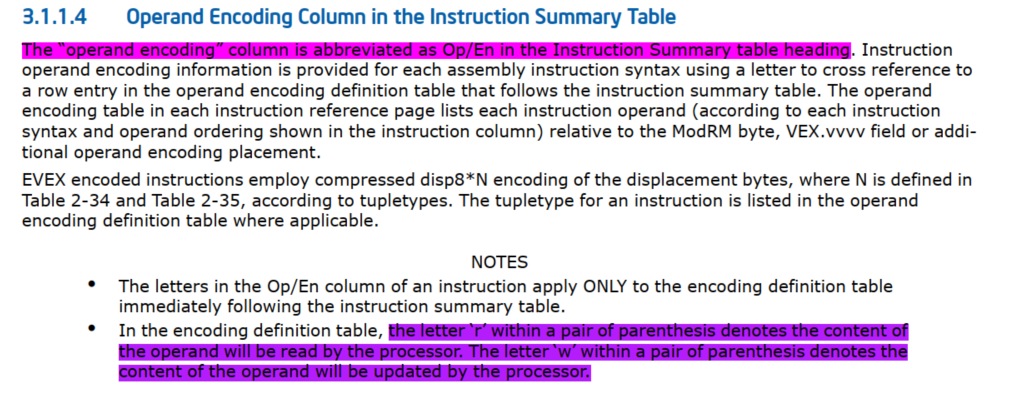1. Background

[base + index*scale + disp]

baseindex是寄存器，disp是偏移量，scale是系数。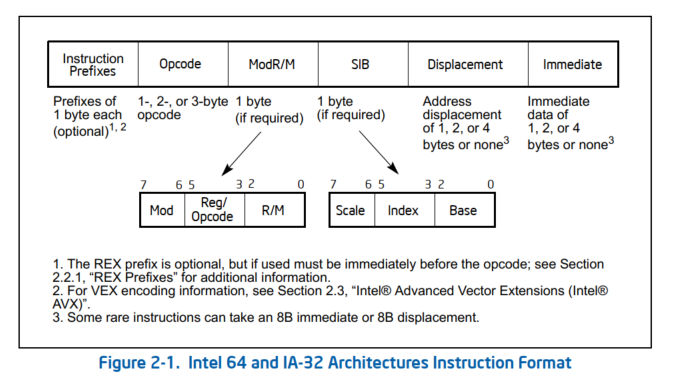Figure1

Figure1中，SIB中的Scale，Index，Base与scale,index,base相对应。 Displacement与disp相对应。

2. Tools and tips for finding out an x86-64 instruction’s encoding

To quickly find out the encoding of an instruction, you can use the GNU assembler as and the objdump tool together. For example, to find out the encoding of the instruction addq 10(%rdi), %r8, you can do it as follows.

First, create a file add.s containing one line

Second, assemble the add.s to object file by

Last, deassemble the object file by

It will print out

Here 4c 03 47 0a is the 4-byte encoding of the addq instruction.

3. Brief introduction to x86-64 instruction encoding

The x86-64 instructions are encoded one by one as a variable number of bytes for each. Each instruction’s encoding consists of:

• an opcode
• a register and/or address mode specifier consisting of the ModR/M byte and sometimes the scale-index-base (SIB) byte (if required)
• a displacement and an immediate data field (if required)

4. An example: manually encode an x86-64 instruction

Let’s take a look at the encoding of an instruction add r8,QWORD PTR [rdi+0xa] (in Intel syntax) in the previous part. Let’s see how it is encoded to 4c 03 47 0a.

From the “add” instruction reference from “ADD”, “INSTRUCTION SET REFERENCE” in the ISA reference Volume 2A., find the line for the encoding of the ADD r64, r/m64 corresponding to this instruction

REX info: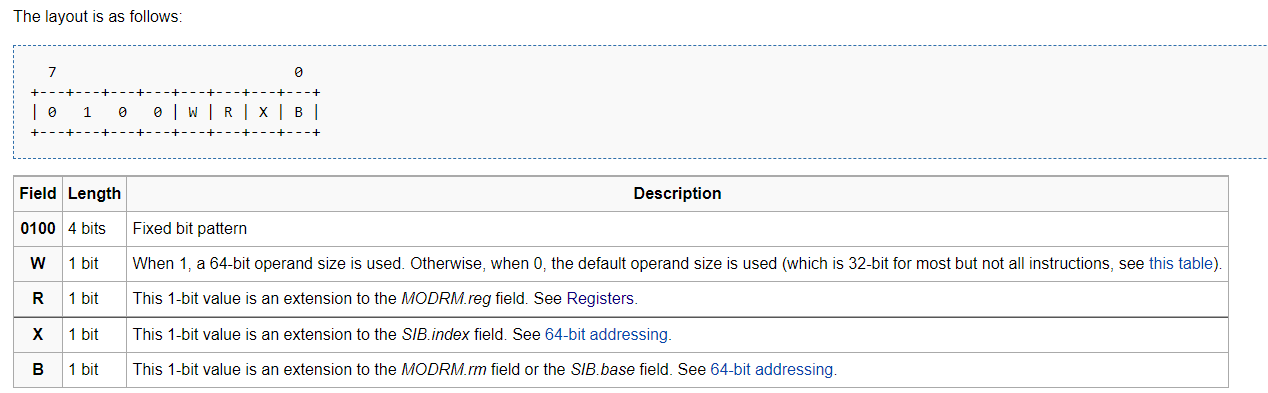and, from the REX description

In 64-bit mode, the instruction’s default operation size is 32 bits. … Using a REX prefix in the form of REX.W promotes operation to 64 bits.

So, we get

The ‘R’, ‘X’ and ‘B’ bits are related to the operand encoding (check “Table 2-4. REX Prefix Fields [BITS: 0100WRXB]” of the reference volume 2A).

REX.X bit modifies the SIB index field.

SIB is not used in this instruction. Hence,

Let’s further look at the encoding of the operands. From the “Instruction Operand Encoding” for the add instruction:

There will be 2 operand parts for the RM encoding. The first part will be ModRM:reg(r,w) and the second part will be ModRM:r/m(r). “Figure 2-4. Memory Addressing Without an SIB Byte; REX.X Not Used” from Volume 2 shows the encoding for this case.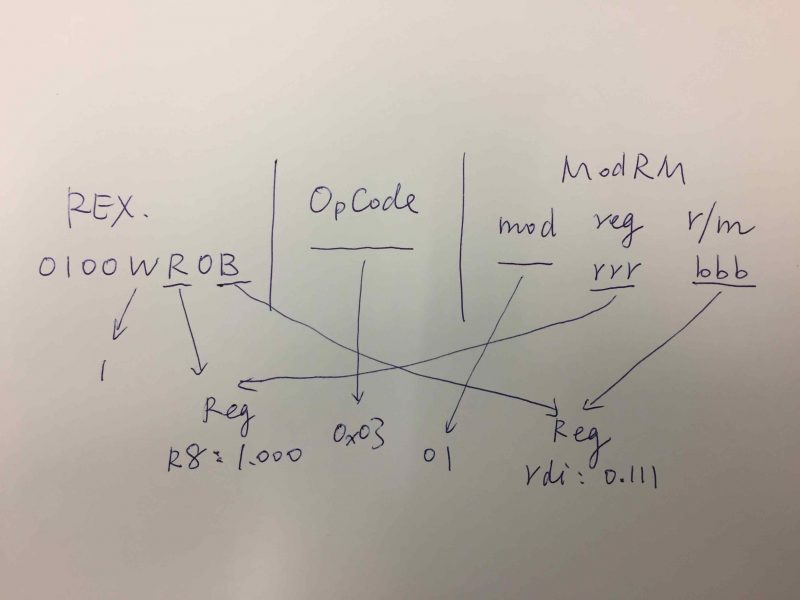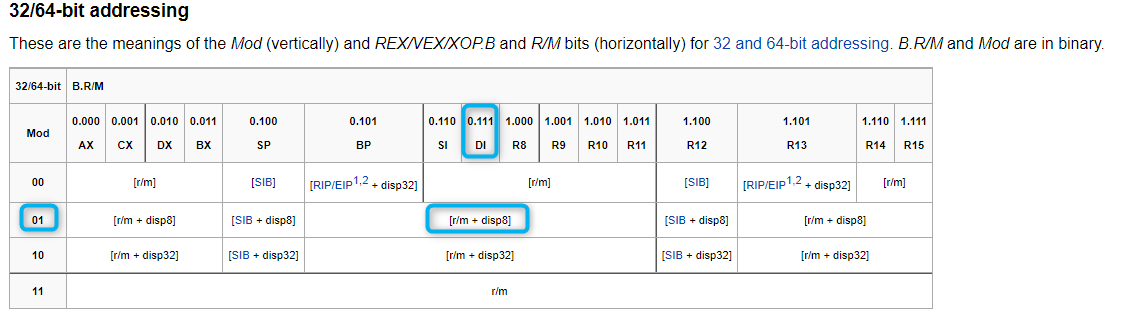The REX.R and REX.B bits and the ModeRM byte will be decided accordingly. There are 3 parts in the ModRM byte: ‘mod’, ‘reg’ and ‘r/m’.

There is a table “Table 2-2. 32-Bit Addressing Forms with the ModR/M Byte” (it is for 32-bit operands. But from 2.2.1.1, “In 64-bit mode, these formats do not change. Bits needed to
define fields in the 64-bit context are provided by the addition of REX prefixes” and hence the same value can be used) in Volume 2 which shows mapping of the operands combinations to the bits values of ‘mod’.

Although the table applies to 64-bit modes too, it does not show the additional registers like r8. Hence, we only use it to find out bits for ‘Mod’ only for the addq instruction we are encoding it. As 0xa can be encoded in a byte, we can use disp8 to keep the instruction encoding short. From the row of [EDI]+disp8 (actually, all disp8 ones share the same ‘Mod’ bits),

For the encoding of the registers, I compiled a table for the general purpose 64-bit registers for your reference:

The ‘_‘ in the ‘_.Reg’ are usually a bit in the REX prefix, such as REX.B and REX.R, depending on specific instructions and operand combinations.

For the addq instruction in this case, r8 is 1.000 and rdi is 0.111. Hence, in bits, we get

Now, let’s put them together.

By putting the ‘WRXB’ bits ([BITS: 0100WRXB]) together, we get the REX prefix for this instruction is

Together with the 03 in REX.W+03/r from the reference for the ADD instruction, the opcode part, in hexadecimal, is

By putting the mod, reg and r/m together, we get the ModRM byte (in bits)

Following the ModRM byte is the displacement is 0xa(10‘s hexadecimal representation) in one byte (disp8).

Putting all these together, we finally get the encoding of add r8,[rdi+0xa]:

In this example, to show the process, I have shown how to manually do an instruction’s encoding which is usually done by the assembler. You may use the same method to encode all other instruction by checking the reference documents for details of every instruction/operand combinations’ cases.

5. Tips

is a very good page from OSDev as a quick reference.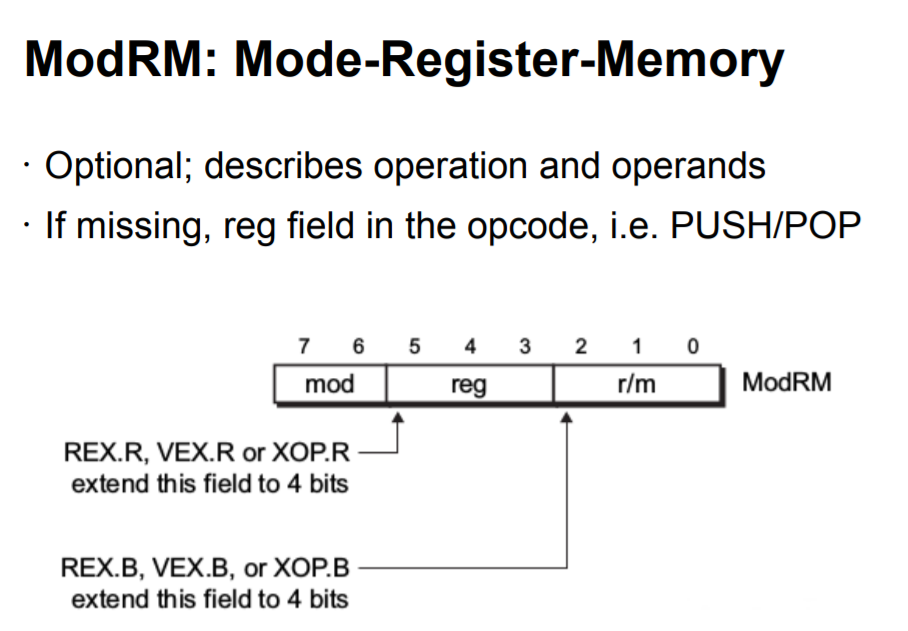• Intel SDM vol2 2.1 INSTRUCTION FORMAT FOR PROTECTED MODE, REAL-ADDRESS MODE,
AND VIRTUAL-8086 MODE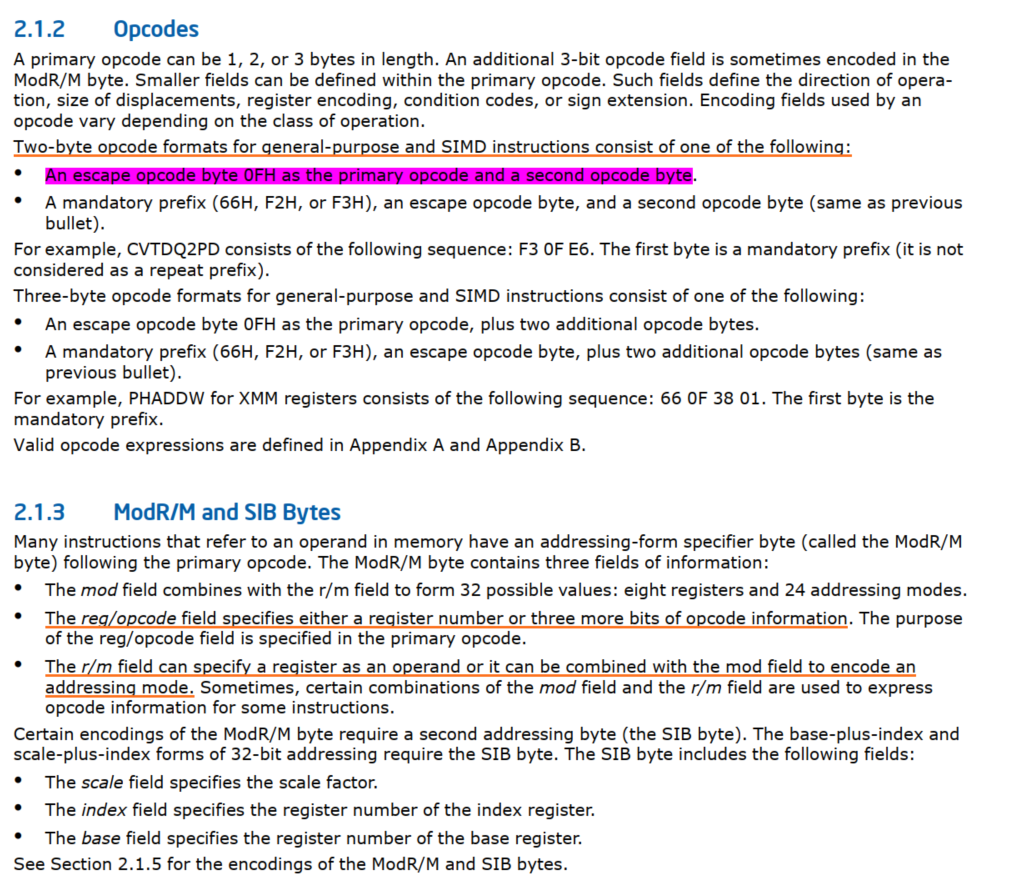• Intel SDM vol2 3.1 INTERPRETING THE INSTRUCTION REFERENCE PAGES

Intel SDM vol2中有具体的指令说明，需要先扫一下3.1 INTERPRETING THE INSTRUCTION REFERENCE PAGES中的内容。This section describes the format of information contained in the instruction reference pages in this chapter. It explains notational conventions and abbreviations used in these sections.

For example: